JEE  >  VITEEE Maths Test - 9

# VITEEE Maths Test - 9

Test Description

## 40 Questions MCQ Test VITEEE: Subject Wise and Full Length MOCK Tests | VITEEE Maths Test - 9

VITEEE Maths Test - 9 for JEE 2023 is part of VITEEE: Subject Wise and Full Length MOCK Tests preparation. The VITEEE Maths Test - 9 questions and answers have been prepared according to the JEE exam syllabus.The VITEEE Maths Test - 9 MCQs are made for JEE 2023 Exam. Find important definitions, questions, notes, meanings, examples, exercises, MCQs and online tests for VITEEE Maths Test - 9 below.
Solutions of VITEEE Maths Test - 9 questions in English are available as part of our VITEEE: Subject Wise and Full Length MOCK Tests for JEE & VITEEE Maths Test - 9 solutions in Hindi for VITEEE: Subject Wise and Full Length MOCK Tests course. Download more important topics, notes, lectures and mock test series for JEE Exam by signing up for free. Attempt VITEEE Maths Test - 9 | 40 questions in 50 minutes | Mock test for JEE preparation | Free important questions MCQ to study VITEEE: Subject Wise and Full Length MOCK Tests for JEE Exam | Download free PDF with solutions
 1 Crore+ students have signed up on EduRev. Have you?
VITEEE Maths Test - 9 - Question 1

### If the maximum value of y = a cos x - 1/3 cos 3x occurs when x = π/6, then the value of a is

VITEEE Maths Test - 9 - Question 2

### The area contained between the curve xy = a2 , the vertical line x = a, x = 4a (a > 0) and x -axis is

VITEEE Maths Test - 9 - Question 3

### If arg (z) = θ, then arg(z̅) =

VITEEE Maths Test - 9 - Question 4

If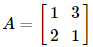then the determinant of A2 - 2A is

VITEEE Maths Test - 9 - Question 5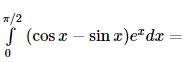VITEEE Maths Test - 9 - Question 6

If z1 and z2 are two non-zero complex numbers such that | z1 + z2 | = | z1 | + | z2 | , then Arg z1 − Arg z2 is

Detailed Solution for VITEEE Maths Test - 9 - Question 6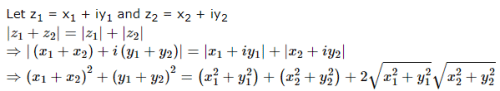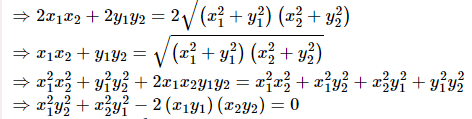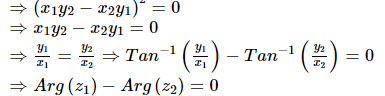VITEEE Maths Test - 9 - Question 7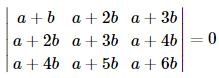VITEEE Maths Test - 9 - Question 8

Let f(x) = log |x - 1|, x ≠ 1. The value of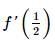is

Detailed Solution for VITEEE Maths Test - 9 - Question 8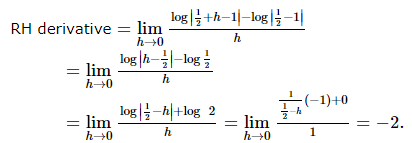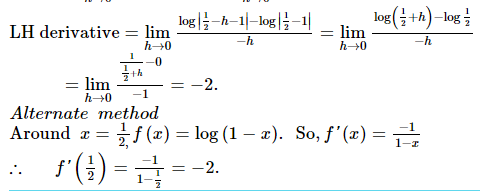VITEEE Maths Test - 9 - Question 9

If f : ℝ → ℝ is defined by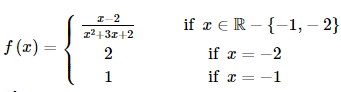then f is continuous on the set

Detailed Solution for VITEEE Maths Test - 9 - Question 9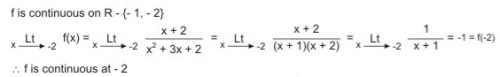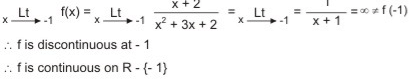VITEEE Maths Test - 9 - Question 10

The solution of the equation cosylog(secx+tanx)dx=cosxlog(secy+tany)dy is

VITEEE Maths Test - 9 - Question 11

The differential equation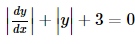admits

VITEEE Maths Test - 9 - Question 12

The differential equation of family of lines which passes (1,-1) is

VITEEE Maths Test - 9 - Question 13

The solution of the equation (dy/dx)=cotx coty is

Detailed Solution for VITEEE Maths Test - 9 - Question 13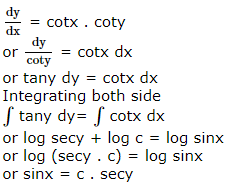VITEEE Maths Test - 9 - Question 14

(d/dx)[tan-1(secx+tanx)]=

VITEEE Maths Test - 9 - Question 15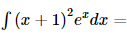VITEEE Maths Test - 9 - Question 16

R is the set of real numbers and f : R → R and g : R are defined by f(x) = 3x2 + 2 and g(x) = 3x - 1 for all x ∈ R. Then

VITEEE Maths Test - 9 - Question 17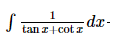Detailed Solution for VITEEE Maths Test - 9 - Question 17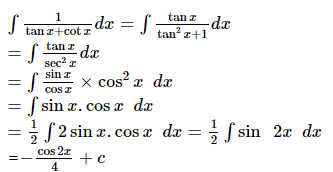VITEEE Maths Test - 9 - Question 18

If cos(2sin-1x)=(1/9), then x=

VITEEE Maths Test - 9 - Question 19

The value of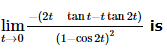VITEEE Maths Test - 9 - Question 20

The value of the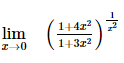is

VITEEE Maths Test - 9 - Question 21

The statement "x > 5 or x < 3" is true if x equals

Detailed Solution for VITEEE Maths Test - 9 - Question 21

For "or" to be true, either (or both) of the conditions must be true.
The only value that makes either of the conditions true is 1.

VITEEE Maths Test - 9 - Question 22

If A and B are two matrices such that AB=B and BA=A, then A2+B2=

VITEEE Maths Test - 9 - Question 23

If A and B are the matrices of same order such that AB = A and BA = B, then A and B are

VITEEE Maths Test - 9 - Question 24

How many words can be formed from the letters of the word COMMITTEE?

VITEEE Maths Test - 9 - Question 25

The probability that a teacher will give an unannounced test during any class meeting is 1/5. If a student is absent twice, then the probability that the student will miss at least one test is

VITEEE Maths Test - 9 - Question 26

Two dice are thrown, the probability that the sum of the points on two dice will be 7 is

VITEEE Maths Test - 9 - Question 27

A sum of money is rounded off to the nearest rupee. The probability that round off error is at least ten paise is

VITEEE Maths Test - 9 - Question 28
If a, b, c, d, e, f are in A.P., then e - c is equal to
VITEEE Maths Test - 9 - Question 29

In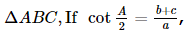, then the triangle is

VITEEE Maths Test - 9 - Question 30

The most stable measure of central tendency is

VITEEE Maths Test - 9 - Question 31

The mean weight of a group of 10 items is 28 and that of another group of n items is 35. The mean of combined group of 10 + n items is found to be 30. The value of n is

VITEEE Maths Test - 9 - Question 32

If one root of the equation x2+px+12=0 is 4, where as the roots of x2+px+q=0 are equal, then the value of q is

VITEEE Maths Test - 9 - Question 33

If the ratio of roots of x2+bx+c=0 and x2+qx+r=0 are equal, then

VITEEE Maths Test - 9 - Question 34

If cos α, cos β, cos γ are the d.c. of a line, then value of sinα2+sin2β+sin2γ is

VITEEE Maths Test - 9 - Question 35
The distance of the point (2,1,-1) from the plane x-2y+4z=9 is
VITEEE Maths Test - 9 - Question 36

If 2cos2x+3sinx-3=0, 0≤x≤1800, then x=

VITEEE Maths Test - 9 - Question 37

The radical centre of the three circles described on the three sides of a triangle as diameter is

VITEEE Maths Test - 9 - Question 38

The lines 2x - 3y = 5 and 3x - 4y = 7 are diameters of a circle having area as 154 sq.units. Then the equation of the cirlce is

VITEEE Maths Test - 9 - Question 39

The unit vector perpendicular to the vector 4i-3j+k in XY-plane is

VITEEE Maths Test - 9 - Question 40

If p=7i-2j+3k and q=3i+j+5k, then the modulus of p-2q is

## VITEEE: Subject Wise and Full Length MOCK Tests

1 videos|3 docs|73 tests
 Use Code STAYHOME200 and get INR 200 additional OFF Use Coupon Code
Information about VITEEE Maths Test - 9 Page
In this test you can find the Exam questions for VITEEE Maths Test - 9 solved & explained in the simplest way possible. Besides giving Questions and answers for VITEEE Maths Test - 9, EduRev gives you an ample number of Online tests for practice

## VITEEE: Subject Wise and Full Length MOCK Tests

1 videos|3 docs|73 tests